Home > CCA2 > Chapter 11 > Lesson 11.1.3 > Problem11-36

11-36.
1. In the casino game of roulette, some players think that when the ball lands on red several times in a row that it will be more likely to land on black on the next spin. You can calculate probabilities to demonstrate that this is not the case. In other words, the outcome of one spin of a roulette wheel does not have any effect on the outcome of the next one. Homework Help ✎

1. Make an area model for two spins of the wheel. Remember that there are 18 red numbers, 18 black numbers, and two greens.

2. What is the probability that the ball will land on red twice in a row?

3. Based on your diagram, what is the probability that the ball will land on red on the second spin?

4. Calculate the conditional probability that the first spin was red given that the second spin is red.

5. How do your answers to parts (c) and (d) compare to the simple probability of the ball landing on red for any single spin? If they are all the same, you have demonstrated that the probability of the ball landing on red is independent of where it landed before.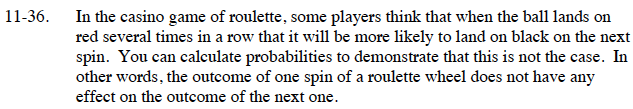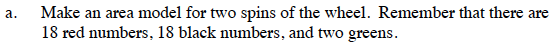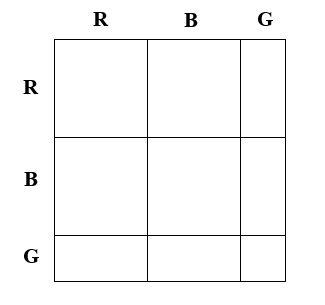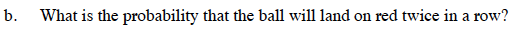Use the diagram you made for part (a).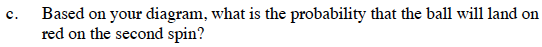Use the diagram you made for part (a).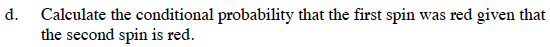Divide the probability you found in part (b) by the probability you found in part (c).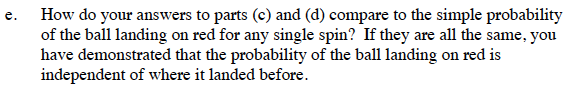If they are not the same, check your work.